## Material Balance for Gas Reservoir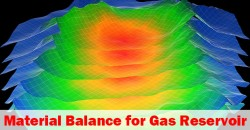For gas reservoirs, the material balance concept can be applied to determine gas in place and expected gas reservoir reserve.

Gas Production = Expansion of Free Gas In Reservoir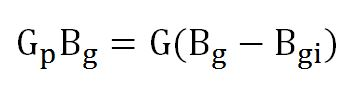Assumption:

• Dry gas reservoir
• No external energy support like water drive.

Where;

Gp = gas production (std cu-ft)

Bg = gas formation volume factor (res cu-ft/std cu-ft)

G = gas in place (std cu-ft)

Bgi = initial formation volume factor (res cu-ft/std cu-ft)

Gas formation volume factor (Bg)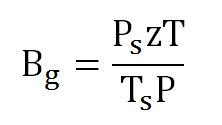Continue reading

## Solution Gas Drive Mechanism Explained in Material Balance Equation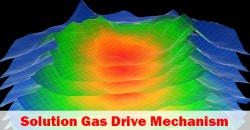Material balance equation can be applied for any drive mechanism and this article demonstrates how to apply the material balance equation in a solution drive mechanism. For a solution drive mechanism, there are 2 cases. The first case is when reservoir pressure is above a bubble point and the second case is when reservoir pressure is below a bubble point.

Solution Gas above Bubble Point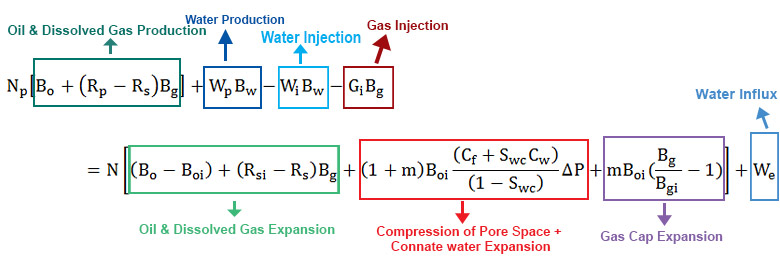Figure 1 – Full Material Balance Equation

## Material Balance Equation in Reservoir EngineeringMaterial balance is a mathematic way to express mass conservation in a reservoir and a simple key principle is “what reservoir is produced must be replaced by other mass.

Volume Produced = Volume Replaced

Volume Produced comes from Gas Production, Oil Production, and Water Production.

Volume Replaced comes from volume expansion, water in flux and water/gas injection.

Figure 1 shows the relationship of the material balance.Figure 1 – Concept of Material Balance

Let’s take a look at each component of equation. Continue reading